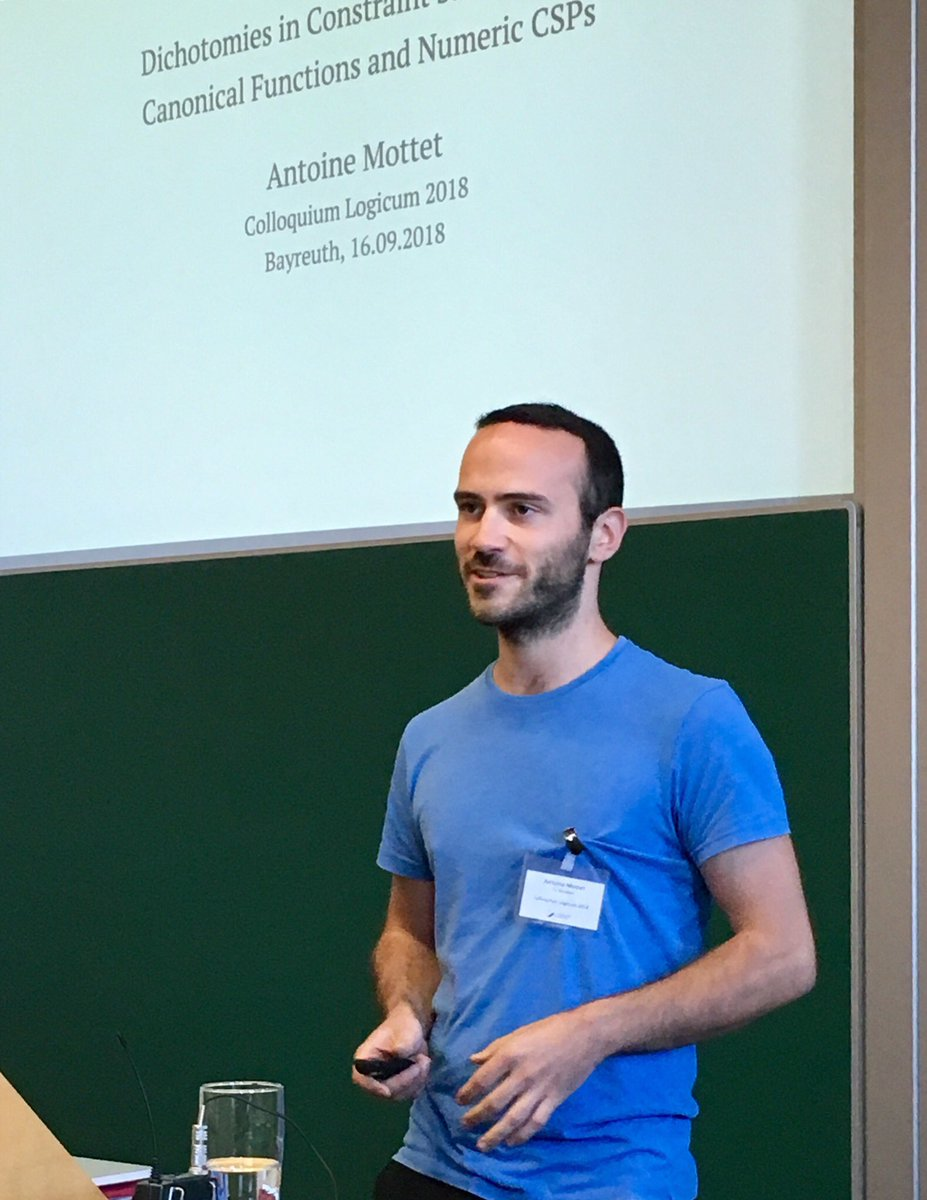# Antoine Mottet

I am interested in applications of algebraic and model-theoretic methods to the study of problems from theoretical computer science, such as the study of constraint satisfaction problems over infinite domains. I am currently assistant professor in the research group on theoretical computer science at the Hamburg University of Technology.

## Selected Papers

• ### Smooth Approximations and CSPs over finitely bounded homogeneous structures

Antoine Mottet, Michael Pinsker.
Proceedings of LICS 2022. Preprint Abstract

We develop the novel machinery of smooth approximations and use it to rederive the known P/NP-complete dichotomy results within the scope of the infinite-domain CSP conjecture. Using the same techniques, we also obtain classification results about CSPs solvable by local consistency methods.

• ### Discrete Temporal Constraint Satisfaction Problems.

Manuel Bodirsky, Barnaby Martin, Antoine Mottet.
In Journal of the ACM 65(2). Abstract

A discrete temporal constraint satisfaction problem is a constraint satisfaction problem (CSP) over the set of integers whose constraint language consists of relations that are first-order definable over the order of the integers. We prove that every discrete temporal CSP is in P or NP-complete, unless it can be formulated as a finite domain CSP, in which case the computational complexity is not known in general.

• ### $\omega$-categorical structures avoiding height 1 identities.

Manuel Bodirsky, Antoine Mottet, Mirek Olšák, Jakub Opršal, Michael Pinsker, Ross Willard.
In Transactions of the AMS 374. Abstract

The algebraic dichotomy conjecture for Constraint Satisfaction Problems (CSPs) of reducts of (infinite) finitely bounded homogeneous structures states that such CSPs are polynomial-time tractable when the model-complete core of the template has a pseudo-Siggers polymorphism, and NP-complete otherwise. One of the important questions related to this conjecture is whether, similarly to the case of finite structures, the condition of having a pseudo-Siggers polymorphism can be replaced by the condition of having polymorphisms satisfying a fixed set of identities of height 1, i.e., identities which do not contain any nesting of functional symbols. We provide a negative answer to this question by constructing for each non-trivial set of height 1 identities a structure whose polymorphisms do not satisfy these identities, but whose CSP is tractable nevertheless. An equivalent formulation of the dichotomy conjecture characterizes tractability of the CSP via the local satisfaction of non-trivial height 1 identities by polymorphisms of the structure. We show that local satisfaction and global satisfaction of non-trivial height 1 identities differ for $\omega$-categorical structures with less than double exponential orbit growth, thereby resolving one of the main open problems in the algebraic theory of such structures.